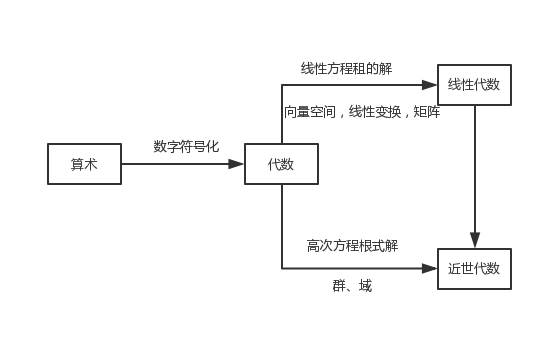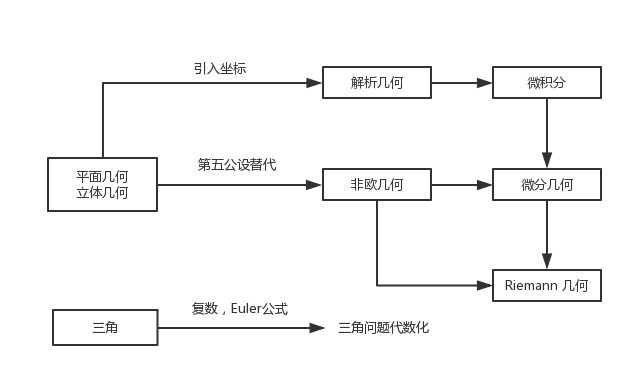# 微积分五讲笔记

## 多变量微积分与单变量微积分的根本差别在于前者有外微分形式

Posted by SHIELD-SKY on January 5, 2018

《微积分五讲》 是华罗庚的弟子，龚昇先生所著，薄薄的一个小本，看完有一种高维打击的感觉

## 数学课程结构## 重点：外微分形式

### 满足下述两条规则的微分乘积称为微分的外乘积：

$\begin{gather} \mathrm dx \wedge \mathrm dx = 0 \\ \mathrm dx \wedge \mathrm dy=- \mathrm dy\wedge \mathrm dx \end{gather}$

Adx Λ dy + Bdy Λ dz + Cdz Λ dx


Hdx Λ dy Λ dz


(1) (λ + μ) Λ ν = λ Λ ν + μ Λ ν
λ Λ (μ + ν) = λ Λ μ + λ Λ ν
(2) λ Λ (μ Λ ν) = (λ Λ μ) Λ ν


(3) 若λ为p次外微分形式，μ为q次外微分形式，则

$\mu \wedge \lambda =\left( -1\right) ^{pq}\lambda \wedge \mu$

$\mathrm df=\dfrac {\partial f}{\partial x}\mathrm dx+\dfrac {\partial f}{\partial y}\mathrm dy + \dfrac {\partial f}{\partial z}\mathrm dz$

$\omega = P\mathrm dx + Q\mathrm dy + R\mathrm dz$

$\mathrm d\omega = \mathrm dP \wedge \mathrm dx + \mathrm dQ \wedge \mathrm dy + \mathrm dR \wedge \mathrm dz$

$\mathrm dP=\dfrac {\partial P}{\partial x}\mathrm dx+\dfrac {\partial P}{\partial y}\mathrm dy + \dfrac {\partial P}{\partial z}\mathrm dz$ $\mathrm dQ=\dfrac {\partial Q}{\partial x}\mathrm dx+\dfrac {\partial Q}{\partial y}\mathrm dy + \dfrac {\partial Q}{\partial z}\mathrm dz$ $\mathrm dR=\dfrac {\partial R}{\partial x}\mathrm dx+\dfrac {\partial R}{\partial y}\mathrm dy + \dfrac {\partial R}{\partial z}\mathrm dz$

$\mathrm d\omega = (\dfrac {\partial P}{\partial x}\mathrm dx+\dfrac {\partial P}{\partial y}\mathrm dy + \dfrac {\partial P}{\partial z}\mathrm dz) \wedge \mathrm dx + (\dfrac {\partial Q}{\partial x}\mathrm dx+\dfrac {\partial Q}{\partial y}\mathrm dy + \dfrac {\partial Q}{\partial z}\mathrm dz) \wedge \mathrm dy +(\dfrac {\partial R}{\partial x}\mathrm dx+\dfrac {\partial R}{\partial y}\mathrm dy + \dfrac {\partial R}{\partial z}\mathrm dz) \wedge \mathrm dz$

$\mathrm d\omega = (\dfrac {\partial R}{\partial y}-\dfrac {\partial Q}{\partial z}) \mathrm dy \wedge \mathrm dz + (\dfrac {\partial P}{\partial z}-\dfrac {\partial R}{\partial x}) \mathrm dz \wedge \mathrm dx +(\dfrac {\partial Q}{\partial x}-\dfrac {\partial P}{\partial y}) \mathrm dx \wedge \mathrm dy$

$\int_{\partial \Omega} \omega = \int_\Omega \mathrm d \omega$

0 直线段 Newton-Leibniz公式
1 平面区域 Green公式
1 空间曲面 Stokes公式
2 空间中区域 Gauss公式